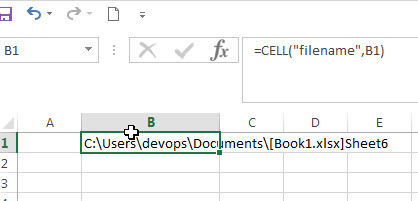# Get full File Name (workbook and worksheet) and Path

In excel, you can get the current workbook name and it is absolute path using the CELL function.  Just refer to the following formula:

`=CELL("filename",B1)`Enter the above formula into any of cells in the current worksheet, then you will get the workbook name and its path, it is similar with the below format:

`path[book1.xlsx]sheet1`

From the result returned by the Cell function, it contain the absolute path of the current workbook, the current workbook name and the current worksheet name.

The second argument of the CELL function is an optional. You can provide one cell reference, then it returns the full information of the worksheet that contain this provided cell. If the cell reference is not specified, then the CELL function will return the full information of the current worksheet.

### Related Formulas

• Get the Current Worksheet Name only
If you want to get the current worksheet name only in excel, you can use a combination of the MID function, the CELL function and FIND function.…
• Get the Current Workbook Name
If you want to get the name of the current workbook only, you can use a combination of the MID function, the CELL function and the FIND Function..…

### Related Functions

• Excel CELL function
The Excel CELL function returns information about the formatting, location, size, or contents of a cell.The syntax of the CELL function is as below:= CELL (info_type,[reference])…
Related Posts

Excel DVAR Function

This post will guide you how to use Excel DVAR function with syntax and examples in Microsoft excel. Description The Excel DVAR Function will get the variance of a population based on a sample of numbers in a column in ...

Excel DSUM Function

This post will guide you how to use Excel DSUM function with syntax and examples in Microsoft excel. Description The Excel DSUM Function will add the numbers in a column or database that meets a given criteria. And so you ...

Excel DSTDEVP Function

This post will guide you how to use Excel DSTDEVP function with syntax and examples in Microsoft excel. Description The Excel DSTDEVP returns the standard deviation of a population based on the entire population of numbers in a column or ...

Excel DSTDEV Function

This post will guide you how to use Excel DSTDEV function with syntax and examples in Microsoft excel. Description The Excel DSTDEV returns the standard deviation of a population based on a sample of numbers in a column or database ...

Excel DMIN Function

This post will guide you how to use Excel DMIN function with syntax and examples in Microsoft excel. Description The Excel DMIN returns the minimum value from a database or a column of a list that matches the specified conditions. ...

Excel DPRODUCT Function

This post will guide you how to use Excel DPRODUCT function with syntax and examples in Microsoft excel. Description The Excel DPRODUCT returns the product of values from a set of records that match criteria that you specify. The DPRODUCT ...

Excel XIRR Function

This post will guide you how to use Excel XIRR function with syntax and examples in Microsoft excel. Description The Excel XIRR function returns the internal rate of return for a series of cash flows that is not necessarily periodic. ...

Excel VDB Function

This post will guide you how to use Excel VDB function with syntax and examples in Microsoft excel. Description The Excel VDB function calculates the depreciation of an asset for a specified period based on the double declining balance method. ...

Excel SYD Function

This post will guide you how to use Excel SYD function with syntax and examples in Microsoft excel. Description The Excel SYD function calculates the sum-of-years’digits depreciation of an asset for a specified period. And it will return a numeric ...

Excel SLN Function

This post will guide you how to use Excel SLN function with syntax and examples in Microsoft excel. Description The Excel SLN function calculates the depreciation of an asset for one period based on the straight line depreciation. And it ...

Sidebar